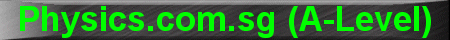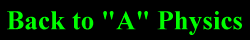(New tips are continually added to these pages.  Check back in a few months' time for more)

TOPIC 13:    Charged Particles

Tip 1:

When a charged particle enters a magnetic field (at right angles to the field), it experiences a force that causes it to move in a circular motion.

This force can be derived from the circular motion equation:

Bqv = mv2/r

But is best memorised as r = mv/Bq.

In this above equation, the velocity of the charged particle is assumed to be constant, from the moment it enters the magnetic field.

Sometimes, students confused this with another situation whereby the charged particle is first accelerated through a potential difference V before entering the magnetic field.

In such a case, the velocity will first have to be determined from the conservation of energy, ie. gain in K.E. = loss in electrical P.E.

˝mv2 = qV

v = Ö(2qV/m)

If we then substitute this expression of v into the equation r = mv/Bq, we get:

r = [mÖ(2qV/m)]/Bq

r = Ö(2mV/B2q)

So, whereas in the first case (velocity assumed to be constant) the radius of the circular motion is proportional to the ratio of m/q; in the second case (when velocity has to be calculated from the accelerating potential) the radius is proportional to Ö(m/q).